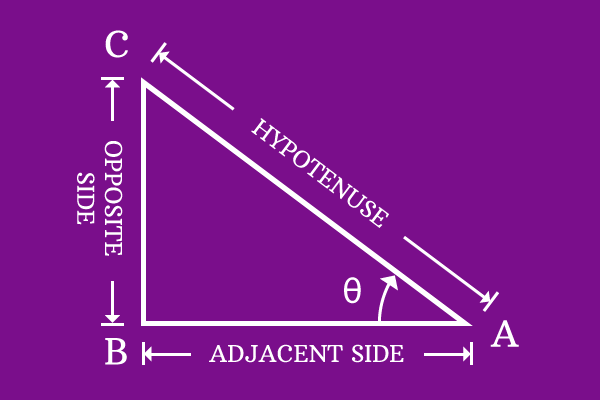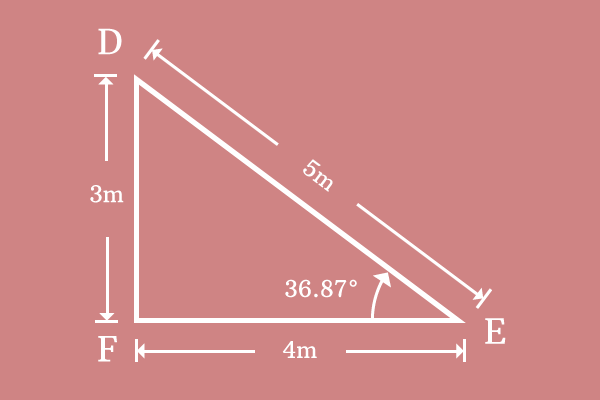# Cotangent

## Definition

A relation of angle with ratio of length of adjacent side to length of opposite side in a right angled triangle is called cotangent.Cotangent represents the relation between angle and the ratio of length of adjacent side to length of opposite side. It is defined in trigonometry from a ratio between two sides. Hence, Cotangent is also called as trigonometric ratio.

Due to the functionality of representing the relation between angle and ratio of length of adjacent side to length of opposite side, Cotangent is also called as trigonometric function in trigonometry.

### Mathematical formIn trigonometry, the relation of angle with ratio of length of adjacent side to length of opposite side is named cotangent. In fact, the variation in angle influences the ratio of length of adjacent side to length of opposite side and vice-versa. The mutual dependency of angle with ratio of length of adjacent side to length of opposite is expressed in mathematics by writing cotangent along with angle but cotangent is simply written in its short form $cot$.

In this case of $Δ BAC$, the angle of right angled triangle is theta $(\theta)$. Therefore, cotangent along with angle is written as $cot \, \theta$ . The relation of angle with ratio of length of adjacent side to length of opposite side is expressed in mathematics as follows.

$$cot \, \theta = {Length \, of \, Adjacent \, side \over Length \, of \, Opposite \, side }$$

In right angled triangle $BAC$, $AB$ and $BC$ are lengths of adjacent side $(\overline{AB})$ and opposite side $(\overline{BC})$. Therefore, express the fundamental expression of cotangent in terms of lengths of adjacent side and opposite side.

$$cot \, \theta = {AB \over BC}$$

#### ExampleConsider $Δ DEF$ to understand the relation between angle and ratio between two sides in trigonometry. The angle and lengths of adjacent side and opposite side are measured as follows.

1. The length of adjacent side $(\overline{EF})$ is $EF = 4$ meters
2. The length of opposite side $(\overline{DF})$ is $DF = 3$ meters
3. The angle of triangle is $36.87^°$

$$cot \, 36.87^° = { EF \over DF }$$

Substitute these three values in this mathematical expression of cotangent.

$$cot \, 36.87^° = {4 \over 3}$$

$⇒ \, cot \, 36.87^° = 1.3333$

Cotangent represents the value of ratio of length of adjacent side to length of opposite side is $1.3333$ at angle $36.87^°$. In other words, the value of cotangent is $1.3333$ at the angle of $36.87^°$. Thus, Cotangent represents the relation of angle with ratio of length of adjacent side to length of opposite side trigonometrically.

Latest Math Topics
Latest Math Problems
Email subscription
Math Doubts is a best place to learn mathematics and from basics to advanced scientific level for students, teachers and researchers. Know more